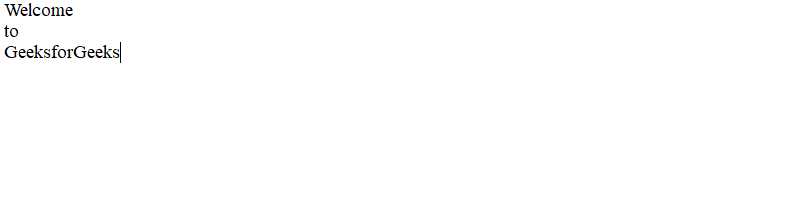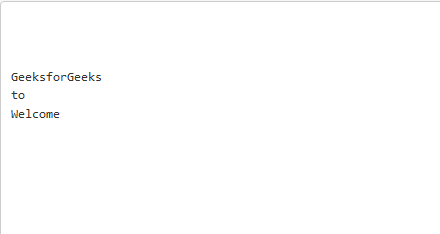GeeksforGeeks App
Open AppBrowser
Continue

# C program to reverse the content of the file and print it

Given a text file in a directory, the task is to print the file content backward i.e., the last line should get printed first, 2nd last line should be printed second, and so on.
Examples:

Input:
file1.txt has:
Welcome
to
GeeksforGeeks
Output:
GeeksforGeeks
to
Welcome
GeeksforGeeks
Input:
file1.txt has:
This is line one
This is line two
This is line three
This is line four
This is line five
Output:
This is line five
This is line four
This is line three
This is line two
This is line one

Approach:

1. Initialise previous length of the text as 0.
2. Find the length of the current line and add it to the previous length. This given the next starting index of the new line.
3. Repeat the above steps till the end of the file.
4. Initialise the array of length of the given message in the given file.
5. Now rewind your file pointer and place the last pointer of the text to arr[K – 1] where K is the length of the array using fseek().
6. Print the length of the last line and decrease K by 1 for printing the next last line of the file.
7. Repeat the above steps until K is equals to 0.

Below is the implementation of the above approach:

## C

 `// C program for the above approach``#include ``#include ``#define MAX 100` `// Function to reverse the file content``void` `reverseContent(``char``* x)``{` `    ``// Opening the path entered by user``    ``FILE``* fp = ``fopen``(x, "a+");` `    ``// If file is not found then return``    ``if` `(fp == NULL) {``        ``printf``("Unable to open file\n");``        ``return``;``    ``}` `    ``// To store the content``    ``char` `buf;``    ``int` `a[MAX], s = 0, c = 0, l;` `    ``// Explicitly inserting a newline``    ``// at the end, so that o/p doesn't``    ``// get effected.``    ``fprintf``(fp, " \n");``    ``rewind``(fp);` `    ``// Adding current length so far +``    ``// previous length of a line in``    ``// array such that we have starting``    ``// indices of upcoming lines``    ``while` `(!``feof``(fp)) {``        ``fgets``(buf, ``sizeof``(buf), fp);``        ``l = ``strlen``(buf);``        ``a = s += l;``    ``}` `    ``// Move the pointer back to 0th index``    ``rewind``(fp);``    ``c -= 1;` `    ``// Print the contents``    ``while` `(c >= 0) {``        ``fseek``(fp, a, 0);``        ``fgets``(buf, ``sizeof``(buf), fp);``        ``printf``("%s", buf);``        ``c--;``    ``}` `    ``return` `;``}` `// Driver Code``int` `main()``{``    ``// File name in the directory``    ``char` `x[] = "file1.txt";` `    ``// Function Call to reverse the``    ``// File Content``    ``reverseContent(x);``    ``return` `0;``}`

Input File:Output File:My Personal Notes arrow_drop_up